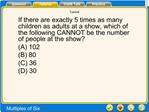DownloadDownload PresentationTutorial

# Tutorial

Télécharger la présentation## Tutorial

- - - - - - - - - - - - - - - - - - - - - - - - - - - E N D - - - - - - - - - - - - - - - - - - - - - - - - - - -
##### Presentation Transcript

1. If there are exactly 5 times as many children as adults at a show, which of the following CANNOT be the number of people at the show? (A) 102 (B) 80 (C) 36 (D) 30 Tutorial

2. If there are exactly 5 times as many children as adults at a show, which of the following CANNOT be the number of people at the show? (A) 102 (B) 80 (C) 36 (D) 30 The total number of people, t, at the show depends on the number of adults, a, plus the number of children, c. Algebraically this can be represented by the equation, t = a + c Tutorial

3. If there are exactly 5 times as many children as adults at a show, which of the following CANNOT be the number of people at the show? (A) 102 (B) 80 (C) 36 (D) 30 We can find some possible values for the total number of people at the show by making an organized table. Tutorial

4. If there are exactly 5 times as many children as adults at a show, which of the following CANNOT be the number of people at the show? (A) 102 (B) 80 (C) 36 (D) 30 If there is only one adult at the show, then there must be five children. Tutorial

5. If there are exactly 5 times as many children as adults at a show, which of the following CANNOT be the number of people at the show? (A) 102 (B) 80 (C) 36 (D) 30 If there are two adults at the show, then there must be ten children. Tutorial

6. If there are exactly 5 times as many children as adults at a show, which of the following CANNOT be the number of people at the show? (A) 102 (B) 80 (C) 36 (D) 30 We can continue to fill in the table in order to determine whether or not a pattern exists for the total number of people at the show. Tutorial

7. If there are exactly 5 times as many children as adults at a show, which of the following CANNOT be the number of people at the show? (A) 102 (B) 80 (C) 36 (D) 30 By examining the columns of the table, let a represent the number of adults at the show. Tutorial

8. If there are exactly 5 times as many children as adults at a show, which of the following CANNOT be the number of people at the show? (A) 102 (B) 80 (C) 36 (D) 30 Tutorial

9. If there are exactly 5 times as many children as adults at a show, which of the following CANNOT be the number of people at the show? (A) 102 (B) 80 (C) 36 (D) 30 Tutorial

10. If there are exactly 5 times as many children as adults at a show, which of the following CANNOT be the number of people at the show? (A) 102 (B) 80 (C) 36 (D) 30 Tutorial

11. If there are exactly 5 times as many children as adults at a show, which of the following CANNOT be the number of people at the show? (A) 102 (B) 80 (C) 36 (D) 30 Tutorial

12. If there are exactly 5 times as many children as adults at a show, which of the following CANNOT be the number of people at the show? (A) 102 (B) 80 (C) 36 (D) 30 Tutorial

13. If there are exactly 5 times as many children as adults at a show, which of the following CANNOT be the number of people at the show? (A) 102 (B) 80 (C) 36 (D) 30 Tutorial